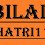Water Quantity Calculation In Rectangular Tank | Learn How To Calculate Water Quantity

# Water Quantity Calculation In Rectangular Tank | Learn How To Calculate Water QuantityWe will explain here how to work out the size and capacity of a water tank with a rectangular or square shape.
Use following formula to find out volume of tank
Volume of tank = Length x Breadth x Height
You can find out quantity of water in 1 cuft in the following ways :-
Density of water = 1000 kg/cum
1 cum = 1000 litres (1000 kg)
1 cum = 35.3198 cuft
Therefore, the quantity of water in 1 cuft = 1000/35.3198 = 28.31 litres
Therefore, weight of water = 576 x 28.31 = 16307 litres = 16307 kg
When the dimension is provided in meter, multiple volume with 1000 to get the amount in liters.
With adherence to IS code, 135 litres is required for each person each day. Split of the IS assumptions :
Drinking – 5 litres
Cooking – 5 litres
Bathing & Toilet – 85 litres
Washing Clothes & Utensils – 30 litres
Cleaning House – 10 litres
Watch the video:

1.2.3.